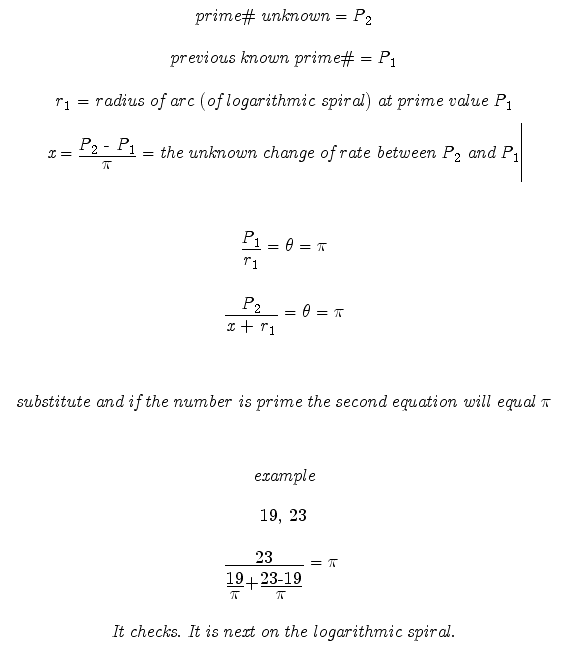_______ 20070605 Theory: How to determine (verify) and predict if a number is Prime: Most all mathematics can be explained graphically. So why should this not be true of prime numbers.---- If a logarithmic spiral is created with each Pi radians * the radius equal to the corresponding sequential prime number---- then the equation of the logarithmic spiral describes the changing of the rate and can be used to determine and verify prime numbers.I will be adding more content to support this work soon. However do not wait and test the theory for yourself. May the Creative Force be with You!